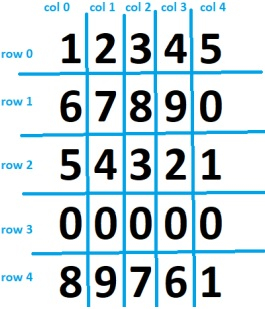# Row-wise vs column-wise traversal of matrix in C++

A matrix can be traversed in two ways. Row-mise traversal visits each row one by one starting from first row then second and so on till the last row. Elements in the row are returned from index 0 to the last index.

In Column-wise traversal, elements are traversed from the first column to the last column in order.

In 2D matrix M[i][j]. Index i is used for representing rows and index j is used for representing columns. For row-wise traversal, start from

i=0th row and 0<=j<last index

i=1st row and 0<=j<last index

.....

i=last row and 0<=j<last index

For column-wise traversal, start from

j=0th column and 0<=i<last index

j=1st column and 0<=i<last index

.....

j=last column and 0<=i<last index

The order of indexes remains same in 2D array M[i][j]- i for rows and j for columns

## Examples

Input

int arr[MAX][MAX] = { {1,2,3,4,5},{6,7,8,9,0},
{5,4,3,2,1},{0,0,0,0,0},
{8,9,7,6,1}};

Output

Row Major Traversal
1 2 3 4 5
6 7 8 9 0
5 4 3 2 1
0 0 0 0 0
8 9 7 6 1
Column Major Traversal
1 6 5 0 8
2 7 4 0 9
3 8 3 0 7
4 9 2 0 6
5 0 1 0 1

Explanation −The output is self-explanatoryInput

int arr[MAX][MAX] = { {1,1,1,1,1},{2,2,2,2,2},
{3,3,3,3,3},{4,4,4,4,4},
{5,5,5,5,5}};

Output

Row Major Traversal
1 1 1 1 1
2 2 2 2 2
3 3 3 3 3
4 4 4 4 4
5 5 5 5 5
Column Major Traversal
1 2 3 4 5
1 2 3 4 5
1 2 3 4 5
1 2 3 4 5
1 2 3 4 5

Explanation − The output is self-explanatory.

## Approach used in the below program is as follows

In this approach we use two for loops for printing the input 2D matrix in row-wise and column wise traversals.

• Take the input array arr[][] for representing 2D matrix.

• Take variables i and j as indexes for row elements and column elements.

• For row-wise traversal start a for loop from index i=0 to i<MAX, for traversing row by row

• Inside it, start a nested for loop from j=0 to j<MAX, for traversing all elements of ith row.

• Print arr[i][j]

• For column-wise traversal start a for loop from index j=0 to j<MAX, for traversing column by column

• Inside it, start a nested for loop from i=0 to i<MAX, for traversing all elements of jth column.

• Print arr[i][j].

## Example

#include <bits/stdc++.h>
using namespace std;
#define MAX 5
int main(){
int arr[MAX][MAX] = { {1,2,3,4,5},{6,7,8,9,0},{5,4,3,2,1},{0,0,0,0,0},{8,9,7,6,1}};
int i, j;
cout<<"Row Major Traversal "<<endl;
for(i=0;i<MAX;i++){
cout<<endl;
for(j=0;j<MAX;j++){
cout<<" "<<arr[i][j];
}
}
cout<<endl<<endl;
cout<<"Column Major Traversal "<<endl;
for(j=0;j<MAX;j++){
cout<<endl;
for(j=0;j<MAX;j++){
cout<<" "<<arr[j][i];
}
}
return 0;
}

## Output

If we run the above code it will generate the following Output

Row Major Traversal
1 2 3 4 5
6 7 8 9 0
5 4 3 2 1
0 0 0 0 0
8 9 7 6 1
Column Major Traversal
6 5 0 8 3 2 7 6 4